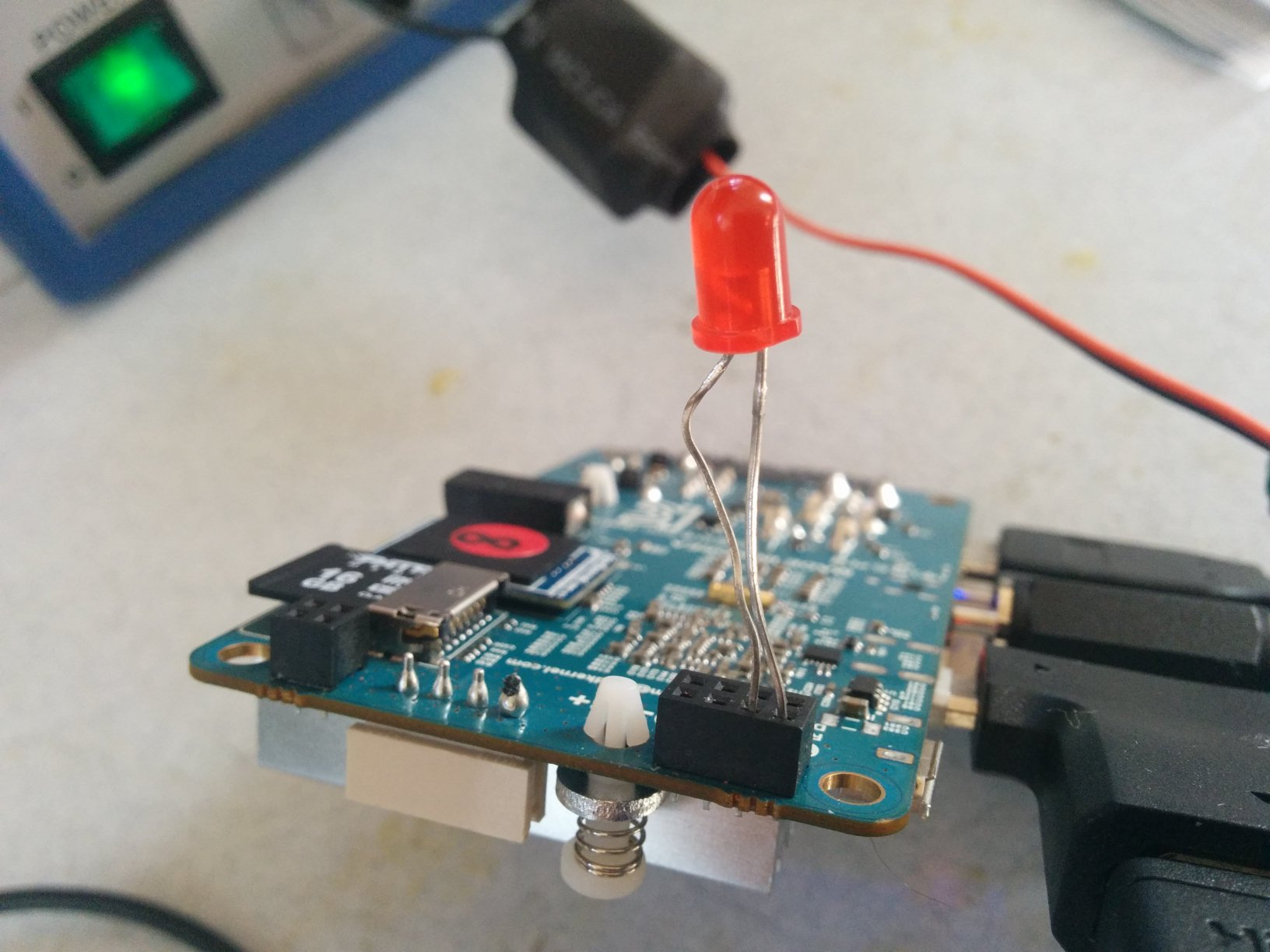Accessing GPIO pins on the Odroid U3+ Linux in Bash/PythonBash Tutorial

To learn how to access the GPIO pins on the Odroid U3+ on a Linux system, I added a red LED to the GPIO pins, and the objective is to light that on. Here are the steps to do that:

1. Connect a low voltage LED, red preferably. As shown in the photo:2. Log in as root using `su -`.

3. Set the pins indicated in the photo above as output:

``````cd /sys/class/gpio
echo 204 > export
cd gpio204
echo out > direction
``````
4. Write a bash loop to light on and off the LED:

``````while true; do
echo 0 > value
sleep 1
echo 1 > value
sleep 1
done
``````

GPIO Schematic

Simple schematic of the GPIOs as viewed from the angle of the photo above:

 1.8V UART RX UART TX 5V GPIO199 GPIO200 GPIO204 GND

It would be great if someone creates a simple python or java library to access these, right?

Edit: I have created the simple python library, it is over at GitHub here:
https://github.com/zaiddabaeen/gpioU3P

Python Tutorial

A simple python library to control the three GPIO pins on the Odroid U3+.

Clone the library using:

``````git clone https://github.com/zaiddabaeeen/gpiou3p
``````

Usage

Simply import the library

``````import gpiou3p as gpio
``````

Writing to the pin

1. Setup the pin directions

``````gpio.setup(gpio.PIN.GPIO199, gpio.DIRECTION.OUT)
``````
2. Write to the pin

``````gpio.write(1, gpio.PIN.GPIO199)
``````

1. Setup the pin directions

``````gpio.setup(gpio.PIN.GPIO199, gpio.DIRECTION.IN)
``````
``````gpio.read(gpio.PIN.GPIO199)
Check `example.py` file for an example case that blinks an LED.